2017-10-18 15:26:18 qq996980215 阅读数 173
• ###### MATLAB图像处理

全面系统的学习MATLAB在图像处理中的应用

20274 人正在学习 去看看 魏伟import java.awt.Graphics;
import java.awt.Image;
import java.awt.image.BufferedImage;
import java.awt.image.ConvolveOp;
import java.awt.image.Kernel;
import java.io.File;
import java.io.IOException;
import java.util.Date;

import javax.imageio.ImageIO;

/**
* javas算子,边缘提取
* @author Bob
* 2017年10月14日15:22:39
*/
public class EdgeExtraction {

/**
* 获取图片的边界轮廓
* @param image
* @return
*/
public static  BufferedImage getImageEdge(BufferedImage image) {
int imageWidth = image.getWidth();
int imageHeight = image.getHeight();
BufferedImage newPic = new BufferedImage(imageWidth, imageHeight,BufferedImage.TYPE_INT_RGB);
float[] elements = { 0.0f, -1.0f, 0.0f,
-1.0f,  4.0f,-1.0f,
0.0f, -1.0f, 0.0f };
Kernel kernel = new Kernel(3, 3, elements);
ConvolveOp cop = new ConvolveOp(kernel, ConvolveOp.EDGE_NO_OP, null);
cop.filter(image, newPic);
return newPic;
}

/**
* 根据所给的算子做处理
* 5.0以上都是不同程度的曝光过度
* 图像锐化（拉普拉斯算子）是5.0，
* 获取图片的边界轮廓  是 4.0，
* @param image 图像
* @param operator 算子
* @return
*/
public static  BufferedImage getImageEdge(BufferedImage image,float operator) {
int imageWidth = image.getWidth();
int imageHeight = image.getHeight();
BufferedImage newPic = new BufferedImage(imageWidth, imageHeight,BufferedImage.TYPE_INT_RGB);
//算子：图像锐化（拉普拉斯算子）中间是5.0，
float[] elements = { 0.0f, -1.0f, 0.0f,
-1.0f,  operator,-1.0f,
0.0f, -1.0f, 0.0f };
Kernel kernel = new Kernel(3, 3, elements);
ConvolveOp cop = new ConvolveOp(kernel, ConvolveOp.EDGE_NO_OP, null);
cop.filter(image, newPic);
return newPic;
}

/**
* 根据所给的算子模板做处理
* @param image 图像
* @param elements 算子模板
* @return
* @throws Exception
*/
public static  BufferedImage getImageEdge(BufferedImage image, float[] elements) throws Exception {
int size=(int)Math.sqrt(elements.length);
if(size*size!=elements.length){
throw new Exception("模板错误,模板长度必须为n*n(n为整形)");
}
int imageWidth = image.getWidth();
int imageHeight = image.getHeight();
BufferedImage newPic = new BufferedImage(imageWidth, imageHeight,BufferedImage.TYPE_INT_RGB);

Kernel kernel = new Kernel(size,size, elements);
ConvolveOp cop = new ConvolveOp(kernel, ConvolveOp.EDGE_NO_OP, null);
cop.filter(image, newPic);
return newPic;
}
/**
* @param args
*/
public static void main(String[] args) {
Image a;
try {
int width=a.getWidth(null);
int height=a.getHeight(null);

BufferedImage image=new BufferedImage(width, height, BufferedImage.TYPE_INT_RGB);
Graphics g=image.getGraphics();
g.drawImage(a, 0, 0, width, height, null);
g.dispose();

ImageIO.write(getImageEdge(image), "png", new File("e:/new_"+new Date().getTime()+".png"));

} catch (IOException e) {
// TODO Auto-generated catch block
e.printStackTrace();
}

}
}


2018-08-11 11:56:00 dbsggal90047018 阅读数 25
• ###### MATLAB图像处理

全面系统的学习MATLAB在图像处理中的应用

20274 人正在学习 去看看 魏伟
Robert算子是一阶微分算子，比较简单，计算量小，对细节反应敏感。
Sobel算子是基于一阶导数的微分算子，其中引入了类似局部平均的运算，对于噪声有平滑作用，能很好地消除噪声的影响，所以计算量变大。
Prewitt算子和Sobel一样，图像中的点用Sobel的两个卷积核卷积，取最大值作为输出值。也增加了计算量。
Canny算子比较复杂，既要计算多个方向的梯度，又要记录方向和大小，然后又进行NMS非极大值抑制，大小阈值，反正很复杂就对了，自己百度一下。

2019-02-27 23:33:14 rocketeerLi 阅读数 209
• ###### MATLAB图像处理

全面系统的学习MATLAB在图像处理中的应用

20274 人正在学习 去看看 魏伟

# 低通滤波

## 邻域平均法

$g(x,y) = \frac{1}{M}\sum_{(i,j)\in S}f(i,j)$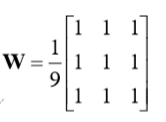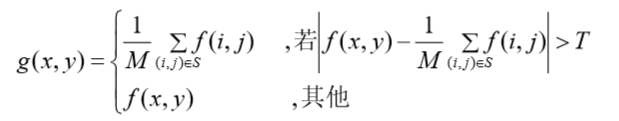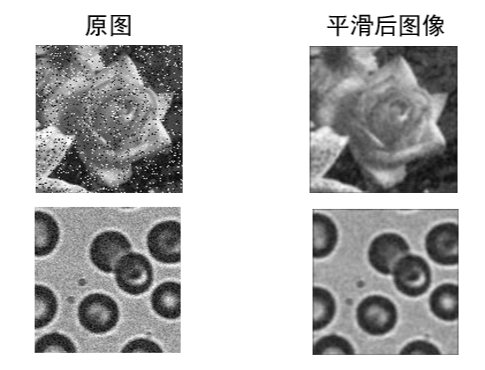$H = \frac1{16}\begin{bmatrix} 1 & 2 & 1 \\ 2 & 4 & 2 \\ 1 & 2 & 1\end{bmatrix}$

## 中值滤波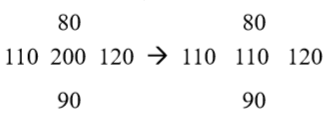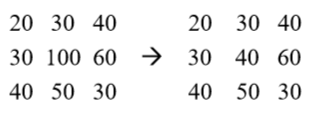# 高通滤波## Roberts算子

Roberts 算子是利用的局部像素差来进行边缘检测。是 2*2 窗口的，利用对角线相邻像素之差来进行检测。矩阵变化如下：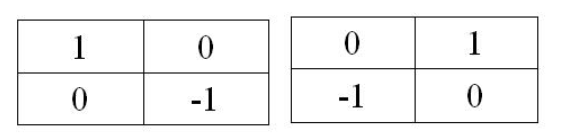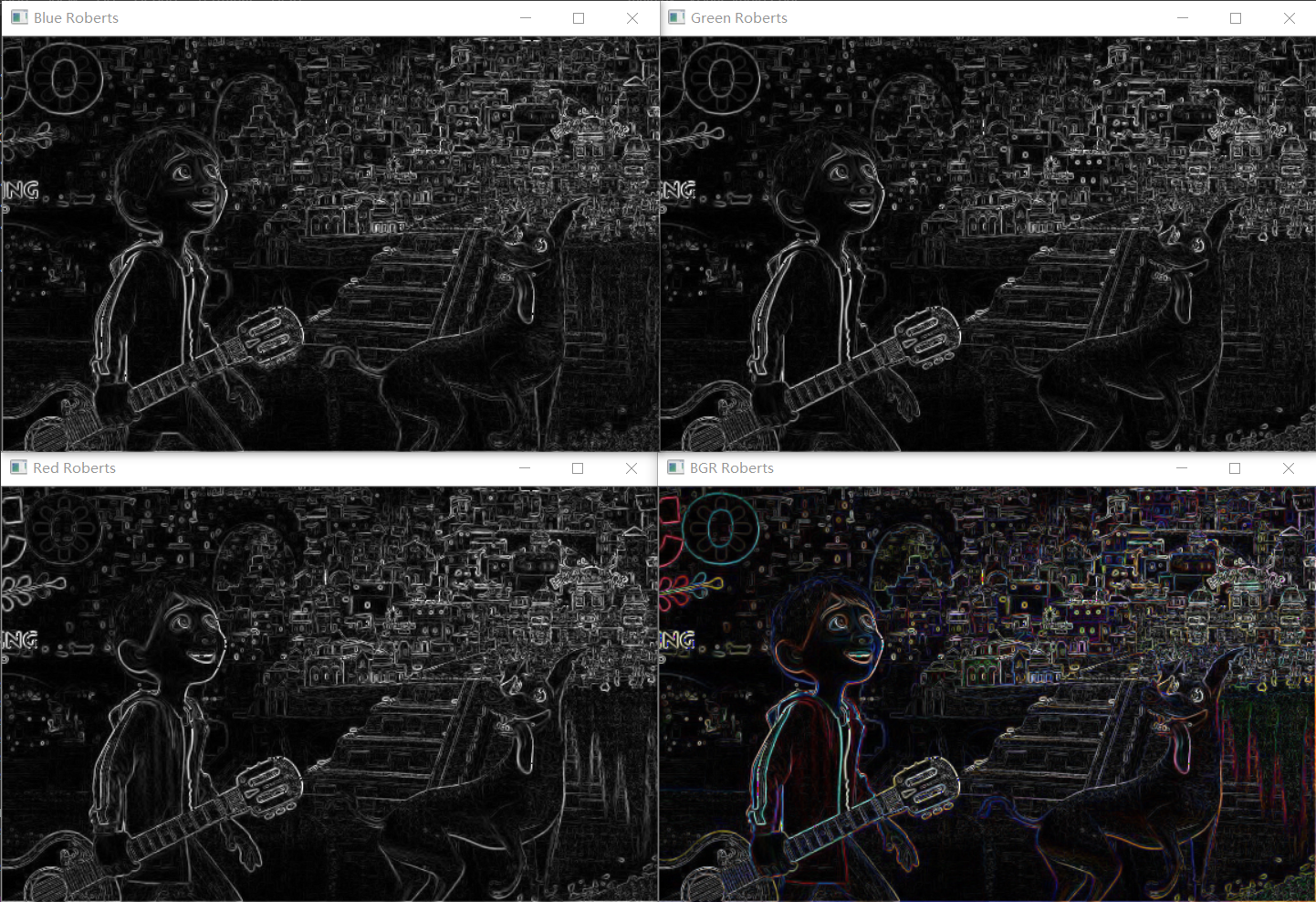## Sobel 算子

Sobel 算子是对阶跃边缘，对数字图像{f(i,j)}的每个像素，考察它上、下、左、右邻点 灰度的加权差。与之接近的邻点权大。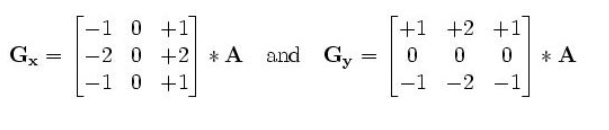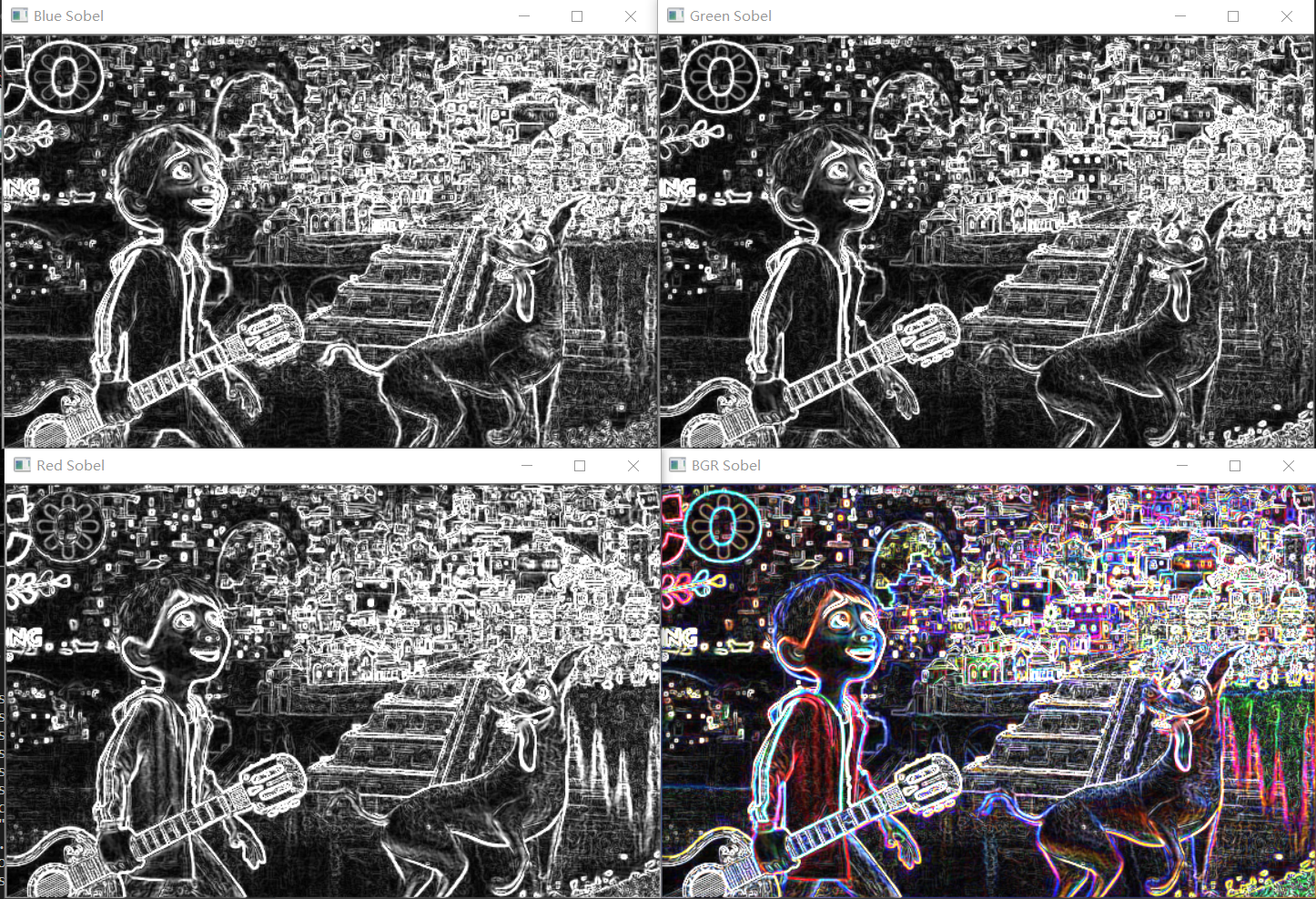# 最后

2016-08-04 17:22:04 u013496193 阅读数 1674
• ###### MATLAB图像处理

全面系统的学习MATLAB在图像处理中的应用

20274 人正在学习 去看看 魏伟2019-10-16 20:22:15 weixin_43262648 阅读数 578
• ###### MATLAB图像处理

全面系统的学习MATLAB在图像处理中的应用

20274 人正在学习 去看看 魏伟

# 数字图像处理——Sobel算子锐化、Prewitt算子锐化

%函数名称为Image_Sobel,输入参数Image,输出参数IMAGE
function [IMAGE] = Image_Sobel(Image)
%获取矩阵的行、列、波段数
[m,n,bands] = size(Image);
%定义模板大小，假设模板大小3×3
A = 1;
%定义Sobel算子x，y方向矩阵
Sobelx = [-1 -2 -1;0 0 0;1 2 1];
Sobely = [-1 0 1;-2 0 2;-1 0 1];
%初始化矩阵
Image1 = zeros(m,n,bands);
IMAGE = Image;
%Sobel算子
for k = 1:bands
for i = 1+A:m-A
for j = 1+A:n-A
temp = Image(i-A:i+A,j-A:j+A,k);
Image1(i,j,k) = abs(sum(sum(Sobelx.*temp)))+abs(sum(sum(Sobely.*temp)));
end
end
end
IMAGE = Image + Image1;
%画图，左右分别表示原图和两幅处理后的图像
figure(1);
subplot(1,3,1);
imshow(uint8(Image));
title('原始图像');
subplot(1,3,2);
imshow(uint8(Image1));
title('边缘提取图像');
subplot(1,3,3);
imshow(uint8(IMAGE));
title('Sobel算子锐化后的图像')
end


%函数名称为Image_Prewitt,输入参数Image,输出参数IMAGE
function [IMAGE] = Image_Prewitt(Image)
%获取矩阵的行、列、波段数
[m,n,bands] = size(Image);
%定义模板大小，假设模板大小3×3
A = 1;
%定义Prewitt算子x，y方向矩阵
Prewittx = [-1 -2 -1;0 0 0;1 2 1];
Prewitty = [-1 0 1;-2 0 2;-1 0 1];
%初始化矩阵
Image1 = zeros(m,n,bands);
IMAGE = Image;
%Sobel算子
for k = 1:bands
for i = 1+A:m-A
for j = 1+A:n-A
temp = Image(i-A:i+A,j-A:j+A,k);
Image1(i,j,k) = abs(sum(sum(Prewittx.*temp)))+abs(sum(sum(Prewitty.*temp)));
end
end
end
IMAGE = Image + Image1;
%画图，左中右分别表示原图和两幅处理后的图像
figure(1);
subplot(1,3,1);
imshow(uint8(Image));
title('原始图像');
subplot(1,3,2);
imshow(uint8(Image1));
title('边缘提取图像');
subplot(1,3,3);
imshow(uint8(IMAGE));
title('Prewitt锐化后的图像')
end


Image = double(imread('当前目录下的图片名称.jpg'));
Sobel = Image_Sobel(Image);
Prewitt = Image_Prewitt(Image);